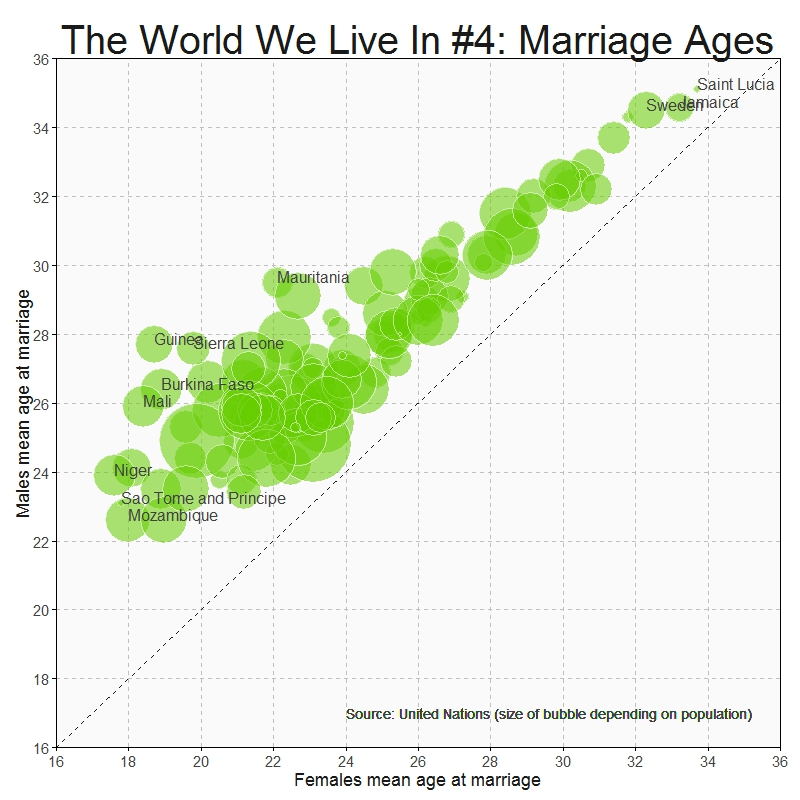# The World We Live In #4: Marriage Ages

It is time for women to stop being politely angry (Leymah Gbowee, Nobel Prize Peace Winner)

Sometimes very simple plots give insight into we live in a world of differences. This plot shows the mean age at marriage for men and women across countries:Being a woman in some countries of this world must be a hard experience:

```#Singulate mean age at marriage: http://data.un.org/Data.aspx?d=GenderStat&f=inID%3a20
#Population: http://data.un.org/Data.aspx?d=SOWC&f=inID%3a105
require("sqldf")
require("ggplot2")
colnames(mar)="Country"
colnames(pop)="Country"
data=sqldf("SELECT
a.Country,
a.Value as Pop,
b.Value as Female,
c.Value as Male
FROM
pop a INNER JOIN mar b
ON (a.Country=b.Country AND b.Subgroup='Female') INNER JOIN mar c
ON (a.Country=c.Country AND c.Subgroup='Male')
WHERE a.Subgroup = 'Total'")
opts=theme(
panel.background = element_rect(fill="gray98"),
panel.border = element_rect(colour="black", fill=NA),
axis.line = element_line(size = 0.5, colour = "black"),
axis.ticks = element_line(colour="black"),
panel.grid.major = element_line(colour="gray75", linetype = 2),
panel.grid.minor = element_blank(),
axis.text = element_text(colour="gray25", size=15),
axis.title = element_text(size=18, colour="gray10"),
legend.key = element_blank(),
legend.position = "none",
legend.background = element_blank(),
plot.title = element_text(size = 40, colour="gray10"))
ggplot(data, aes(x=Female, y=Male, size=log(Pop), label=Country), guide=FALSE)+
geom_point(colour="white", fill="chartreuse3", shape=21, alpha=.55)+
scale_size_continuous(range=c(2,36))+
scale_x_continuous(limits=c(16,36), breaks=seq(16, 36, by = 2), expand = c(0, 0))+
scale_y_continuous(limits=c(16,36), breaks=seq(16, 36, by = 2), expand = c(0, 0))+
geom_abline(intercept = 0, slope = 1, colour = "gray10", linetype=2)+
labs(title="The World We Live In #4: Marriage Ages",
x="Females mean age at marriage",
y="Males mean age at marriage")+
geom_text(data=subset(data, abs(Female-Male)>7), size=5.5, colour="gray25", hjust=0, vjust=0)+
geom_text(data=subset(data, Female>=32|Female<=18), size=5.5, colour="gray25", hjust=0, vjust=0)+
geom_text(aes(24, 17), colour="gray25", hjust=0, label="Source: United Nations (size of bubble depending on population)", size=5)+opts
```